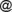16-17 May 2019 • Sofia, Bulgaria

Submission: 21 February 2019Notification: 11 March 2019Final Version: 1 April 2019

# Issue:Trapezoidal/triangular intuitionistic fuzzy numbers versus interval-valued trapezoidal/triangular fuzzy numbers and applications to multicriteria decision making methods

Jump to: navigation, search
 shortcut http://ifigenia.org/wiki/issue:nifs/20/2/43-51
Title of paper: Trapezoidal/triangular intuitionistic fuzzy numbers versus interval-valued trapezoidal/triangular fuzzy numbers and applications to multicriteria decision making methods
Author(s):
 Adrian Ban Department of Mathematics and Informatics, University of Oradea, Universităţii 1, 410087 Oradea, Romania aibanuoradea.ro Delia Tuşe Department of Mathematics and Informatics, University of Oradea, Universităţii 1, 410087 Oradea, Romania dmercauoradea.ro
Presented at: 18th International Conference on Intuitionistic Fuzzy Sets, 10–11 May 2014, Sofia, Bulgaria
Published in: "Notes on IFS", Volume 20, 2014, Number 2, pages 43—51
Download:
Abstract: We establish relationships between the set of trapezoidal intuitionistic fuzzy numbers and the set of interval-valued trapezoidal fuzzy numbers and, on the other hand, between the set

of triangular intuitionistic fuzzy numbers and the set of triangular fuzzy numbers. Based on these main results of the paper, the methods or procedures elaborated for interval-valued trapezoidal or triangular fuzzy numbers as input data can be easy transferred to the case of trapezoidal or triangular intuitionistic fuzzy numbers as input data. We exemplify by transferring an interval-valued trapezoidal multicriteria decision making method in a trapezoidal intuitionistic fuzzy method.

Keywords: Trapezoidal/triangular fuzzy number, Trapezoidal/triangular intuitionistic fuzzy number, Interval-valued trapezoidal/triangular fuzzy number.
AMS Classification: 03E72, 62C86
References:
1. Atanassov, K. T., Intuitionistic fuzzy sets, Fuzzy Sets and Systems, Vol. 20, 1986, 87-96.
2. Atanassov, K. T., Intuitionistic Fuzzy Sets: Theory and Applications, Springer Physica–Verlag, Heidelberg, 1999.
3. Ban, A., O. Ban, Optimization and extensions of a fuzzy multicriteria decision making method and applications to selection of touristic destinations, Expert Systems with Applications, Vol. 39, 2012, 7216–7225.
4. Ban, A., Approximation of intuitionistic fuzzy numbers by trapezoidal fuzzy numbers preserving the expected interval, Advances in Fuzzy Sets, Intuitionistic Fuzzy Sets, Generalized Nets and Related Topics. Volume I: Foundations (Atanassov, K., O. Hryniewicz, J. Kacprzyk, M. Krawczak, E. Szmidt, Eds.), Academic House Exit, Warszawa, 2008, 53–83.
5. Ban, A., L. Coroianu, Approximate solutions preserving parameters of intuitionistic fuzy linear systems, Notes on Intuitionistic Fuzzy Sets, Vol. 17, 2011, 58–70.
6. Ban, A., L. Coroianu, P. Grzegorzewski, Trapezoidal approximation and aggregation, Fuzzy Sets and Systems, Vol. 177, 2011, 45–59.
7. Ban, A., L. Coroianu, Simplifying the search for effective ranking of fuzzy numbers, IEEE Transactions on Fuzzy Systems, DOI 10.1109/TFUZZ.2014.2312204 (to appear).
8. Chen, S. J., S. M. Chen, Fuzzy risk analysis based on similarity measures between intervalvalued fuzzy numbers, Computers and Mathematics with Applications, Vol. 55, 2008, 1670–1685.
9. Chen, S. M., J. H. Chen, Fuzzy risk analysis based on similarity measures between intervalvalued fuzzy numbers and interval-valued fuzzy number arithmetic operators, Expert Systems with Applications, Vol. 36, 2009, 6309–6317.
10. Deschrijver, G., E. Kerre, On the relationship between some extensions of fuzzy set theory, Fuzzy Sets and Systems, Vol. 133, 2003, 227–235.
11. Heilpern, S., The expected value of a fuzzy number, Fuzzy Sets and Systems, Vol. 47, 1992, 81–86.
12. Hong, D. H., S. Lee, Some algebric properties and a distance measure for interval-valued fuzzy numbers, Information Sciences, Vol. 148, 2002, 1–10.
13. Li, D. F., A ratio ranking method of triangular intuitionistic fuzzy numbers and its application to MADM problems, Computers and Mathematics with Applications, Vol. 60, 2010, 1557–1570.
14. Xu, Z., Sh. Shang, W. Qian, W. Shu, A method for fuzzy risk analysis based on the new similarity of trapezoidal fuzzy numbers, Expert Systems with Applications, Vol. 37, 2010, 1920–1927.
15. Yao, J. S., F. T. Lin, Constructing a fuzzy flow-shop sequencing model based on statistical data, International Journal Approximate Reasoning, Vol. 29, 2002, 215–234.
Citations:

The list of publications, citing this article may be empty or incomplete. If you can provide relevant data, please, write on the talk page.